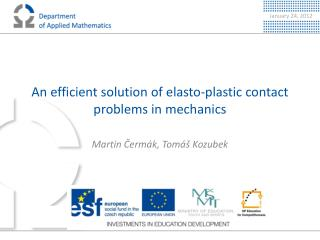Download PresentationAn efficient solution of elasto -plastic contact problems in mechanics

# An efficient solution of elasto -plastic contact problems in mechanics

Download Presentation## An efficient solution of elasto -plastic contact problems in mechanics

- - - - - - - - - - - - - - - - - - - - - - - - - - - E N D - - - - - - - - - - - - - - - - - - - - - - - - - - -
##### Presentation Transcript

1. An efficient solution of elasto-plastic contact problems in mechanics Martin Čermák, Tomáš Kozubek

2. Contents • Elasto-plastic problem • Total-FETI domain decomposition method • Numerical experiment • Future work • References

3. Elasto-plastic problem • quasi-static problems, where the history of loading is taken into account • von Miseselasto-plasticity with the strain isotropic hardening • finite element method with the return mapping concept • the elasto-plastic deformation of an body after loading • The Cauchy stress tensor • The small strain tensor • The displacement • The nonnegative hardening parameter

4. Elasto-plastic law 1/2 Constitutive model • , where

5. Elasto-plastic law 2/2 Time discretisation • Implicit Euler • incremental method Theequation of equilibrium

6. Return mappingconcepts

7. Algorithm 1. (initial step) for (time steps) set (zero approximation) do build variables: by Algorithm 2. solve linear system: update displacement: iteration increase: while update end (for cycle over time steps)

8. Algorithm 2. • Input variables are For every elements Compute (area in 2D or volume in 3D) (trial stress update) (yield function update) If then (elastic predictor) Else (return mapping corrector) End Save local variables with subscript into output variables End • Output variables:

9. F Total-FETI domain decomposition • Main ideas: • All subdomains are free with the same defect • Kernels of all subdomain stiffness matrices are known apriori • Generalized inverse is computed effectively by the Choleskifactorization[4,5,6] 4) T. Kozubek et al. : Total FETI domain decomposition method and its massively parallel implementation, Computers and Structures, 2011, submitted. 5) Z. Dostál, et al. : Choleskydecompositionof a positive semidefinite matrix with known kernel. Accepted for publishing inA.M.C. 6) T. Brzobohaty, et al. : Cholesky–SVD decomposition with fixing nodes to stable computation of ageneralized inverse of the stiffness matrix of a floating structure. Accepted forpublishing in I.J.N.M.E.

10. TFETI for equality constrains • Primal formulation: subject to • Saddle-point formulation: • Substitution: • Dual formulation: , where • Solver: PCGP

11. TFETI for inequality constrains • Primal formulation: subject to , • Solver: SMALSE-M with MPGP   Z. Dostál, T. Kozubek: An optimal algorithm and superrelaxation for minimization of a quadratic function subject to separable convex constraints with applications. Accepted for publishing in Mathematical Programming, 2010.

12. Modification of Algorithm 1 for TFETI with equality constrains (initial step) for (time steps) set (zero approximation) Do for each subdomain build variables: and save into end solve problem: subject to by algorithm PCGP update displacement: iteration increase: while update end (for cycle over time steps)

13. Modification of Algorithm 1 for TFETI with inequality constrains (initial step) for (time steps) set (zero approximation) do for each subdomain build variables: and save into end update contact conditions solve problem: subject to , by algorithm SMALSE-M update displacement: iteration increase: while update end (for cycle over time steps)

14. Numerical experiments in 2D • Pressure, is applied to the upper side of (boundary ) • The elasto-plastic material parametrs • E = 206900[MPa] • ν = 0.29 • = 450 • The time interval [0,] [sec] is divided into 50 steps • Consider a mesh with 13122 nodes and 25600 triangles. • Stopping criterion of the Newton method : • Stopping criterion of the SMALSE-M algorithm  : .

15. Plastic and elastic elements for Hertz in 2D Plastic and elasticelements after 50 timesteps Plastic and elasticelements after25 timesteps

16. Hertz 2D - results Von Mises stress distribution on thedeformedmesh Total displacement

17. Numerical experiments in 3D • Pressure, is applied to the upper side of (boundary) • The elasto-plastic material parametersare thesameas in thepreviousbenchmark • Consider a mesh with 3456nodes and 2000 hexahedrons. • Stopping criterion of the Newton method : • Stopping criterion of the SMALSE-M algorithm : .

18. Plastic and elastic elements for Hertz in 3D Plastic and elasticelements after 50 timesteps Plastic and elasticelements after25 timesteps

19. Hertz 3D - results Von Mises stress distribution on thedeformedmesh Total displacement

20. Future work • Finish the parallel implementation of elasto-plastic problem with contact. • Add friction • Finish my Ph.D. work

21. References • Z. Dostál: Optimal Quadratic Programming Algorithms, with Applications to Variational Inequalities, 1st edition, SOIA 23, Springer US, New York, 2009. • Z. Dostál, T. Kozubek, A. Markopoulos, T. Brzobohatý, V. Vondrák, P. Horyl : Theoretically supported scalable TFETI algorithm for the solution of multibody 3D contact problems with friction, CMAME, 2011, DOI: 10.1016/j.cma.2011.02.015. • Z. Dostál, T. Kozubek: An optimal algorithm and superrelaxation for minimization of a quadratic function subject to separable convex constraints with applications. Mathematical Programming, 2011. • T. Kozubek V. Vondrák, M. Menšík, D. Horák,Z. Dostál, V. Hapla, P. Kabeliková, M. Čermák: Total FETI domain decomposition method and its massively parallel implementation, Computers and Structures, 2011, submitted. • R. Blaheta: Numerical methods in elasto-plasticity, DocumentaGeonica 1998, PERES Publishers, Prague, 1999. • S. Sysala: Application of the Modified Semismoth Newton Method to Some Elasto-plastic Problems Mathematics and Computer in Simulation, Modelling 2009.

22. Thank you for your attention.